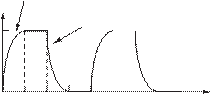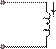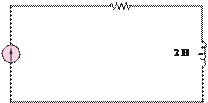### Create an Account

Already have account?

### Forgot Your Password ?

Home / Questions / 428 Use the defining law for an inductor to find the current iLt corresponding to the volt...

# 428 Use the defining law for an inductor to find the current iLt corresponding to the voltage shown in Figure P428 Sketch your result Figure

4.28 Use the defining law for an inductor to find the current iL(t) corresponding to the voltage shown in Figure P4.28. Sketch your result.

Figure P4.24L

(t) = 0.5 - 0.5e-t/0.02

4.25      In the circuit shown in Figure P4.25, let

⎧ 0                    for −∞ 0

 i(t) =

⎪⎨ t                     for 0 ≤ 1s

(− 2)      for1s ≤ 2s

 ⎪

⎩ 0                    for2s ≤

Find the energy stored in the inductor for all time.

vL(t)

0

0.1

0.2      0.3

(t) = 0.5-0.5(t-0.3)/0.02

 L

0.4

(s)

+

vL(t)

_iL(t) 1 H1 Ù

Jun 19 2020 View more View Less

#### Answer (Solved)Subscribe To Get Solution